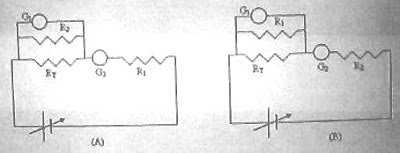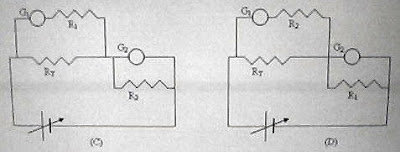## Wednesday, May 19, 2010

### IIT-JEE 2010 – Multiple Choice Questions (Straight Objective Single Correct Answer Type) on Direct Current Circuits

Today we will discuss three multiple choice questions which appeared in IIT-JEE 2010 question paper:(1) Consider a thin square sheet of side L and thickness t, made of a material of resistivity ρ. The resistance between two opposite faces, shown by the shaded areas in the figure is

(A) directly proportional to L

(B) directly proportional to t

(C) independent of L

(D) independent of t

We have R = ρL/A where A is the cross section area which is equal to L t.

Therefore, R = ρL/Lt = ρ/t, which is independent of L [Option (C)].

(2) Incandescent bulbs are designed by keeping in mind that the resistance of their filament increases with increase in temperature. If at room temperature, 100 W, 60 W and 40 W bulbs have filament resistances R100, R60 and R40 respectively, the relation between these resistances is :

(A) 1/R100 = 1/R40 + 1/R60

(B) R100 = R40 + R60

(C) R100 > R60 > R40

(D) 1/R100 > 1/R60 > 1/R40

The power P dissipated in a resistance R is given by

P = V2/R where V is the voltage applied across the resistance.

This shows that the resistance of the filament is smaller if the wattage of the incandescent lamp is greater. In other words, the reciprocal of the filament resistance is greater if the wattage of the incandescent lamp is greater. This is true at all temperatures including room temperature. Therefore we have

1/R100 > 1/R60 > 1/R40

(3) To verify Ohm's law, a student is provided with a test resistor RT, a high resistance R1, a small resistance R2, two identical galvanometers G1 and G2, and a variable voltage ource V. The correct circuit to carry out the experiment isTo verify Ohm’s law, the current through RT is to be measured by connecting an ammeter in series with it and the voltage across RT is to be measured by connecting a voltmeter in parallel with it. One galvanometer is to be converted into an ammeter by connecting the small resistance R2 across it. The other galvanometer is to be converted into a voltmeter by connecting the high resistance R1 in series with it. So the correct circuit to carry out the experiment is the one shown in option (C).

You will find some multiple choice questions (with solution) on direct current circuits here.